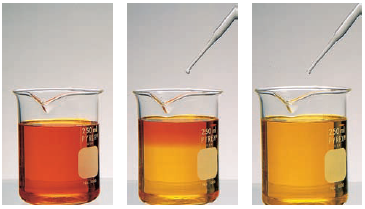# Problem: Chromium(VI) forms two different oxyanions, the orange dichromate ion, Cr 2O72-, and the yellow chromate ion, CrO42-. (See the following photos.) The equilibrium reaction between the two ions isCr2O7 2-(aq) + H2O(l) ⇌ 2CrO4 2-(aq) + 2 H+(aq)Explain why orange dichromate solutions turn yellow when sodium hydroxide is added.

🤓 Based on our data, we think this question is relevant for Professor Young's class at UCSD.

###### FREE Expert Solution

Cr2O7 2-(aq) + H2O(l) ⇌ 2 CrO4 2-(aq) + 2 H+(aq)

• NaOH will react with H+

• NaOH + H+ → H2O + Na+

H+ is being consumed → H+ is a product in the equilibrium reaction###### Problem Details

Chromium(VI) forms two different oxyanions, the orange dichromate ion, Cr 2O72-, and the yellow chromate ion, CrO42-. (See the following photos.) The equilibrium reaction between the two ions is

Cr2O7 2-(aq) + H2O(l) ⇌ 2CrO4 2-(aq) + 2 H+(aq)

Explain why orange dichromate solutions turn yellow when sodium hydroxide is added.